# Numerical Study of the Solidification of Phase Change Materials in a Rectangular Cavity: Effects of Convection and Aspect Ratio

Numerical Study of the Solidification of Phase Change Materials in a Rectangular Cavity: Effects of Convection and Aspect Ratio
Laboratoire de Recherche sur la Physico-chimie des Surfaces et Interfaces, Université de Skikda, Skikda 21000, Algérie

Département de Physique, Université Mohamed Seddik Benyahia, Jijel 18000, Algérie

Corresponding Author Email:
e.mezaache@univ-skikda.dz
Page:
1-9
|
DOI:
https://doi.org/10.18280/acsm.430101
15 November 2018
|
Accepted:
2 February 2019
|
Published:
25 April 2019
| Citation

OPEN ACCESS

Abstract:

The purpose of this study is to investigate the influence of the aspect ratio and Grashof number on the convection diffusion phenomena associated with solid liquid phase transition processes during PCM solidification within a rectangular cavity. The applied two-dimensional physical model is based on the enthalpy method approach. The numerical solutions are obtained with finite volume method and validated with existing numerical and experimental studies of natural convection in phase change material. Numerical analysis are performed using water as phase change material, for an aspect ratio range 0.5 to 4. The left and right walls of the cavity are maintained at hot and cold temperatures, respectively, while the upper and lower surfaces are considered adiabatic. From this study, it is found that PCM initially liquid evolves to thermal stationary regime where solid and liquid regions are present. The solidification phase change process depends considerably on the thermal and geometrical parameters of the system. Additionally, it is demonstrated that the physical approach based on a purely conductive model remains valid to cases of low values of Grashof number and aspect ratio. The findings of this research may contribute to the elaboration of an efficient modeling of PCM heat storage systems.

Keywords:

heat storage, phase change material, enthalpy method, heat exchanger, liquid solide interface

1. Introduction

Phase change materials (PCMs) have an important role in energy efficiency as a means of optimizing energy-intensive industrial processes. They are encountered in various industrial processes particularly in metallurgy: solidification and melting of pure metals and alloys; in electronics industries: component manufacturing; in food industry: drying and packaging; in habitat: heating, cooling, thermal insulation and storage of heat and cold.

Gupta et al.  presented computational predictions and experimental measurements of the dynamic behavior and performance of an active thermal storage system for domestic applications, based on the use of a hydrated salt phase change material (PCM) and a conventional cylindrical storage tank. The thermal storage (heating) and extraction (cooling) rates for this PCM-filled tank are compared to a water-filled tank.

Talati and Taghilou  simulated the solidification of phase change materials (PCM) within a rectangular finned container. It was shown that the maximum required time for the solidification process of phase change materials inside the container occurs when the container aspect ratio equals 0.5.

Elnajjar  investigated numerically the thermal analysis of a brick containing an organic phase change material (PCM) under a hot climate. The results demonstrate that choosing the proper PCM material was the most important aspect for a successful application, and at least a seven-day analysis period was required.

Esapoura et al.  investigated numerically melting and solidification processes of phase change material embedded in metallic porous foam in a multi-tube heat exchanger under local non-equilibrium thermal condition. The authors studied the effects of number of inner tubes, their arrangement as well as porosity of metallic foam on thermal characteristics of heat storage unit.

Sheikholeslami  employed the finite element method with adaptive mesh to simulate the solidification process of nano enhanced phase change material in existence of radiative heat transfer. The results indicate that solidification rate enhances by dispersing CuO nanoparticles in to water.

Zarajabad and Ahmadi  performed a numerical investigation on a freezer cabin equipped with different PCM thicknesses to explore the thermal behavior of freezer during passive operation. The results show that the increase of PCM thickness, the maintaining time of freezer cabin in standard condition with using cold storage system is enhanced.

In the home comfort, Gorzin et al.  presented an investigation on the charging process of a phase change material (PCM) integrated in a domestic hot water system. Enthalpy porosity method is applied for modeling the phase change process. The results show that by distributing the PCM mass in a specific arrangement in which 40% of PCM mass is in inner tube, 52% reduction in melting time is achieved hence solar energy can be used more efficiently in the time of maximum solar radiation.

Zheng et al.  utilized the copper foam to enhance the thermal performance of the paraffin. The authors discussed the effect of the heating position on the thermal performance of copper foam/paraffin composite phase change material (CPCM). The experimental results showed that the melting time of the CPCM was 20.5 % shorter than that of pure paraffin, which indicted adding copper foam could reduce the thermal resistance inside paraffin.

Solidification of PCMs inside capsules of different geometries has been studied by using a one-dimensional conduction model  and two-dimensional conduction model [10, 11]. These two approaches models are efficient for small capsules under applied uniform cooling surface condition. However, in the case of large cavity submitted to non-uniform cooling surface condition, the natural convection play an important role in the heat and momentum transfer and on the shape of liquid-solid interface. The present investigation can be considered as an extension of the conduction model to take into account of the effect of natural convection on the solidification of PCMs inside cavity. The enthalpy method with finite control volume approach is used. As well as, the present study deals with the influence of the Grashof number characterizing the flow heat transfer by natural convection in liquid region and the aspect ratio factor of the cavity during the solidification of PCMs inside a rectangular enclosure.

The paper is structured as follows: Section 2 describes the physical model. Section 3 presents the mathematical formulation followed by section 4 which describes the numerical method used for the computation, the mesh study and the validation of the present code with experimental and numerical data of the literature. The numerical results are discussed in Section 5. Finally, concluding remarks are presented in Section 6.

2. Physical System

The considered physical system is a rectangular cavity with an aspect ratio A. The enclosure contains a phase change material characterized by a Prandtl number Pr. The vertical walls of the enclosure are isothermal and subjected to hot Th and cold Tc temperatures, respectively, which surround the melting temperature Tm (Tc<Tm<Th). In addition, the two horizontal walls are adiabatic (Figure 1).

## fig.1.png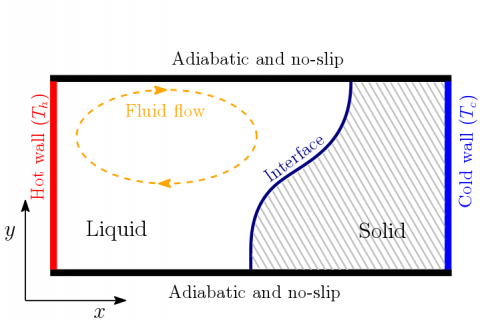Figure 1. Schematic of the physical problem

3. Mathematical Formulation

3.1 Simplifying hypotheses

1. The flow is two-dimensional.
2. The fluid is Newtonian.
3. The physical properties of solid and liquid phases are constant.
4. The viscous dissipation is negligible.
5. The Boussinesq approximation is valid: the density is assumed to be constant in all terms of momentum equations, except in the gravity term, where it is expressed by

$\rho ={{\rho }_{0}}\left[ 1-\beta \text{(}T-{{T}_{r}}\text{)} \right]$   (1)

3.2 Enthalpy method

This method, applied in the present study, is well adapted to the determination of the position of the solid-liquid interface, the knowledge of the physical domains occupied by each of the liquid and solid phases, as well as the kinetics of the solidification. It is described in detail in the literature .

3.3 Dimensionless equations

Reference quantities

${{L}_{r}}=L,\,\,\,\,{{V}_{r}}=\frac{\nu }{{{L}_{r}}},\,\,\,\,\,{{t}_{r}}=\frac{{{L}_{r}}}{{{V}_{r}}},\,\,\,\,\,{{p}_{r}}=\rho V_{r}^{2}$ (2)

$\text{ }\!\!\Delta\!\!\text{ }{{T}_{r}}={{T}_{h}}-{{T}_{c}},\,\,\,\,\text{ }\!\!\Delta\!\!\text{ }{{h}_{r}}={{h}_{h}}-{{h}_{c}}$ (3)

Dimensionless variables

$X=\frac{x}{{{L}_{r}}},Y=\frac{y}{{{L}_{r}}},\tau =\frac{t}{{{t}_{r}}},U=\frac{u}{{{V}_{r}}},V=\frac{\upsilon }{{{V}_{r}}}$ (4)

$\theta =\frac{T-{{T}_{c}}}{\Delta {{T}_{r}}},\hbar =\frac{h-{{h}_{c}}}{\Delta {{h}_{r}}},P=\frac{p}{{{p}_{r}}}$ (5)

Dimensionless numbers

$Gr=\frac{_{\rho g\beta \left( {{T}_{h}}-{{T}_{c}} \right){{\text{H}}^{3}}}}{{{\nu }^{2}}},\Pr =\frac{_{\nu }}{{{\alpha }_{l}}},K=\frac{_{{{k}_{s}}}}{{{k}_{l}}},C=\frac{{{c}_{s}}}{{{c}_{l}}}$ (6)

Governing equations

$\frac{\partial U}{\partial X}+\frac{\partial V}{\partial Y}=0$ (7)

$\frac{\partial U}{\partial \tau }+\frac{\partial \left( UU \right)}{\partial X}+\frac{\partial \left( UV \right)}{\partial Y}=-\frac{\partial P}{\partial X}+\left( \frac{{{\partial }^{2}}U}{\partial \ {{X}^{2}}}+\frac{{{\partial }^{2}}U}{\partial \ {{Y}^{2}}} \right)+{{S}_{U}}$ (8)

\begin{align} & \frac{\partial V}{\partial \tau }+\frac{\partial \left( VU \right)}{\partial X}+\frac{\partial \left( VV \right)}{\partial Y}=-\frac{\partial P}{\partial Y}+\left( \frac{{{\partial }^{2}}V}{\partial \ {{X}^{2}}}+\frac{{{\partial }^{2}}V}{\partial \ {{Y}^{2}}} \right) \\ & \,\,\,\,\,\,\,\,\,\,\,\,\,\,\,\,\,\,\,\,\,\,\,\,\,\,\,\,\,\,\,\,\,\,\,\,\,\,\,\,\,\,\,\,\,\,\,\,\,\,\,\,\,\,\,\,\,\,+Gr\theta +{{S}_{V}} \\\end{align} (9)

\begin{align} & \frac{\partial \hbar }{\partial \tau }+\frac{\partial \left( U\hbar \right)}{\partial X}+\frac{\partial \left( V\hbar \right)}{\partial Y}=\frac{C}{\Pr }\left[ \frac{\partial }{\partial X}\left( K\frac{\partial \hbar }{\partial \ X} \right)+\frac{\partial }{\partial Y}\left( K\frac{\partial \hbar }{\partial \ Y} \right) \right] \\ & \,\,\,\,\,\,\,\,\,\,+\frac{C}{\Pr }\left\{ \frac{\partial }{\partial X}\left[ K\frac{\partial \left( {{\hbar }_{s}}-\hbar \right)}{\partial \ X} \right]+\frac{\partial }{\partial Y}\left[ K\frac{\partial \left( {{\hbar }_{s}}-\hbar \right)}{\partial \ Y} \right] \right\} \\\end{align} (10)

where the Darcy source terms SUand SVare represented respectively by the Carman-Kozeny equations :

${{S}_{U}}=-\zeta \frac{{{\left( 1-{{f}_{l}} \right)}^{2}}}{\left( {{f}_{l}}^{3}+\varepsilon \right)}U$ (11)

${{S}_{V}}=-\zeta \frac{{{\left( 1-{{f}_{l}} \right)}^{2}}}{\left( {{f}_{l}}^{3}+\varepsilon \right)}V$ (12)

$\zeta =\frac{\xi \text{ }\!\!~\!\!\text{ }}{{{\rho }_{0}}}\frac{{{H}^{2}}}{{{v}_{l}}}$ (13)

where the quantities ξ and ε have an important numerical utility in the region when the control volume is occupied by the solid phase (fl 0). The value chosen for ξ is very large to cancel the velocity in solid region, however the value chosen for ε is very small to avoid division by zero. The values used by different authors are ξ=108 kg/m3.s and ε=0-3 [13-15].

Based on the enthalpy method, the liquid fraction fl is expressed by:

${{f}_{l}}=\left\{ \begin{matrix} 0 & {} & h\le {{c}_{s}}{{T}_{m}} \\ \frac{\left( h-{{c}_{s}}{{T}_{m}} \right)}{\ell } & \text{if} & {{c}_{s}}{{T}_{m}}<h<{{c}_{s}}{{T}_{m}}+\ell \\ 1 & {} & h\ge {{c}_{s}}{{T}_{m}}+\ell \\ \end{matrix} \right.\begin{matrix} \text{(solid phase)} \\ \text{(interface)} \\ \text{(liquid phase)} \\\end{matrix}$ (14)

3.4 Initial and boundary conditions

For t=0:

$U=0,V=0,\theta =1\,,\ldots \ldots \hbar =1$ (15)

For t>0:

$X=0\,\text{:}U=0,V=0,\theta =1,\hbar =1$ (16)

$X=1\,\text{:}U=0,V=0,\theta =0,\hbar =0$ (17)

$Y=0\,\text{:}U=0,V=0,\frac{\partial \theta }{\partial Y}=0,\frac{\partial \hbar }{\partial Y}=0$ (18)

$Y=1\,\text{:}U=0,V=0,\frac{\partial \theta }{\partial Y}=0,\frac{\partial \hbar }{\partial Y}=0$ (19)

3.5 Nusselt number

The local Nusselt number Nu and the average Nusselt number $\overline{Nu}$ for the hot and cold walls are defined by:

- Hot wall:

\begin{align} & Nu={{\left. -\frac{\partial \theta }{\partial X} \right|}_{X=0}} \\ & \overline{Nu}=\int\limits_{0}^{1}{Nu\,dY} \\\end{align} (20)

- Cold wall:

\begin{align} & Nu={{\left. -\frac{\partial \theta }{\partial X} \right|}_{X=L}} \\ & \overline{Nu}=\int\limits_{0}^{1}{Nu\,dY} \\\end{align} (21)

4. Numerical Procedure and Validation

In this study, the adopted mathematical model for PCM explores the phenomenon of natural convection in the liquid and the conduction in the solid by a system of coupled partial differential equations, elliptic and nonlinear. The finite volume method is used in this study. The conservation equations (7)-(10) are expressed locally in an integral form. One of the main advantages of this method is to ensure accurate full-size integral conservation whatever the size of the mesh. It also allows a correct treatment of heterogeneous environments and facilitates the linearization of source terms [13-17].

About this work, a program was developed in Fortran language. The results of the calculations are validated numerically and experimentally with previous works. The structure of the flow and its thermal field were represented by contours of streamlines, enthalpies, isotherms and velocity.

The dimensionless equations (7)-(10) of the mathematical model show the dependence of the problem to the Grashof number Gr and Prandtl number Pr. The graphical representation is obtained using graphic software: Tecplot 2014 and Origin 6.0. About the calculation the program was executed on a microcomputer having a processor 3,3 GHz Intel core i5 and 8Gb of RAM.

4.1 Mesh effects

To show the effect of mesh adaptation on numerical solutions, four (04) meshes 98×42, 122×42, 164×64 and 184×64 nodes are tested, for Gr=3.6×105 and rectangular cavity with an aspect ratio equal to 1. The variation of velocity component V at the respective position Y=0.5, achieved in steady state regime, has been presented in Figure 2. It is noted that an identical appearance for the four meshes is shown and the optimal mesh to visualize the solidification phenomenon and to control the solid-liquid interface is 164×64 nodes. This mesh is used in this study and the considered time step (∆τ) is 10-5.

## fig.2.png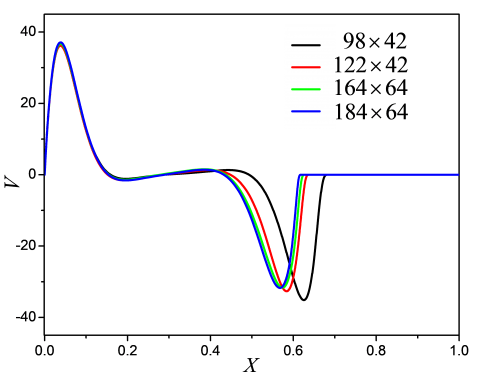Figure 2. Vertical velocity V at Y=0.5 for Gr=3.6×105

4.2 Validation with the experimental data

To validate the calculation program, our results are compared with experimental data obtained by Beckermann and Viskanta  concerning the solidification of pure materials, where the interface is isothermal. A square enclosure with rigid walls is considered, filled with pure gallium. The gallium properties used for validation are presented in Table 1.

Table 1. Physical properties of pure gallium 

 Properties of the PCM Symbol Solid (s) Liquid (l) Unit Heat capacity c 381,5 381,5 J/kg K Thermal conductivity k 32 32 W/m K Density ρ 6,093 103 6,093 103 kg/m3 Kinematic viscosity v 2.97 10-7 2.97 10-7 kg/m s Coefficient of thermal expansion β 6.734×10-5 K-1 Prandtl number Pr 0,0208 Solidification temperature Tm 302.78 K Latent heat ℓ 8,016 103 kJ/kg Hot wall temperature Th 312,98 K Cold wall temperature Tc 297,98 K Height of the cavity H 0.01 m

The consideration of Cerimele et al.  has been taken into account: the following physical properties are considered constants in liquid and solid phases (ρl=ρs=ρ, kl=ks=k and cl=cs=c).

Figure 3 present a comparison of temperature profiles at positions Y=0.133, Y=0.5 and Y=0.867 for non-dimensional time: τ =1.782 (t=10 min) and τ=8.91 (t=50 min). It is found that the numerical results are in good agreement with the experimental results of Beckermann and Viskanta . It is also noted that in the central region at Y=0.133, a slight difference can be seen between the results of this comparison.

## fig.3.pngFigure 3. Comparison of dimensionless temperature θ with experimental data of Beckermann and Viskanta  for (a) t=10 min, (b) t=50 min

4.3 Validation with numerical results

The numerical comparisons concern the solidification of a PCM material. The aluminum, initially liquid at a temperature T0 greater than its melting temperature Tm is considered. The cavity is rectangular, with an aspect ratio A=4. The vertical walls are isothermal and subjected to hot Th and cold Tc temperatures respectively, which surround the melting temperature Tm (Tc<Tm<Th). In addition, the horizontal walls are adiabatic. Aluminum properties are given in Table 2.

Figure 4 represent a comparison, in steady state flow regime, of dimensionless profiles, of streamlines, temperature and isoenthalpic lines, for a Grashof number Gr=8.5×104. A satisfactory agreement with the numerical results of Bouabdallah  and simulations results of present code are observed.

Table 2. Physical properties of aluminum 

 Properties of the PCM Symbol Solid (s) Liquid (l) Unit Heat capacity c 1,066 1,086 kJ/kg K Thermal conductivity k 209 92 W/m K Density ρ 2570 2359 kg/m3 Kinematic viscosity v - 4,92×10-7 kg/m s Coefficient of thermal expansion β - 4×10-5 K-1 Prandtl number Pr - 0.014 Solidification temperature Tm 933 K Latent heat ℓ 397 kJ/kg Hot wall temperature Th 1000 K Cold wall temperature Tc 925 K

## fig.4.pngFigure 4. Comparison of streamlines (a), isotherms (b) and isenthalpic lines (c) between the present result and numerical results  for Gr=8.5×104

5. Results and Discussions

The purpose of the numerical study is to determine the solidification front and visualize the natural convection flow during the solidification of a phase change material contained in a rectangular cavity. The solidification front, the isotherms, and the stream function are determined in terms of Grashof number, characterizing the liquid phase convective heat transfer. The calculation conditions are: Tc=-10 oC, Tm=0 oC, Th=10 oC. The physical properties are given in Table 3. Initially, the enclosure is maintained at a temperature greater than melting temperature (T0>Tm). ie, equal to the temperature of the hot surface. This initial equilibrium state undergoes a temporal evolution by imposing the thermal boundary conditions outside the lateral walls of the rectangular cavity (Tc<Tm<Th). The physical system then evolves towards a new state of equilibrium reflecting the stability of the system which is called the steady state. The discussion and analysis of the results was developed for a domain described by a rectangular cavity.

5.1 Effect of Grashof number

The solidification front and the streamlines are determined and illustrated according to the Grashof number characterizing the flow in the liquid phase.

The considered physical system is initially maintained at a temperature above the melting temperature (T0>Tm). This initial equilibrium state undergoes temporal evolution to a new state of equilibrium by imposing the thermal boundary conditions (Tc<Tm<Th). The physical system reaches a new

equilibrium reflecting its steady state. The structure and the thermal behavior of the flow were represented by contours of the streamlines, isoenthalpies and isotherms.

Figure 5 showed that for the dominant conduction case, assumed to be the ideal case, the isotherms intercepted the horizontal walls as straight lines in parallel. It is similar for the case of the distribution of isoenthapies at the solid-liquid interface, notable by a thickness of the narrower interface.

The structure of temperature coupled with non-dimensional streamlines is illustrated in Figure 6.

Table 3. Physical properties of water 

 Properties of the PCM Symbol Solid (s) Liquid (l) Unit Heat capacity c 2.06 4,182 kJ/kg K Thermal conductivity k 2.26 0.6 W/m K Density ρ 916.8 998.8 kg/m3 Dynamic viscosity μ - 1,2×10-6 kg/m s Kinematic viscosity V - 1.0032×10-6 m2/s Thermal diffusivity a - 1.435×10-7 m2/s Coefficient of thermal expansion β - 6.734×10-5 K-1 Prandtl number Pr - 6.9 Solidification temperature Tm 273.15 K Latent heat ℓ 335 kJ/kg Hot wall temperature Th 283 K Cold wall temperature Tc 263 K

## fig.5.png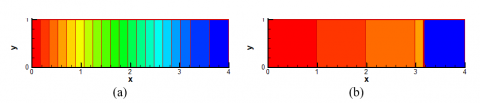Figure 5. Isotherms (a) and isoenthalpy (b) at Gr=0

## fig.6.pngFigure 6. Temperature distribution coupled with streamlines for different Grashof number

For the contours of the stream function an apparent unicellular structure for the Grashof number, Gr=105, whose maximum φmax=2.2 is centrally located in the liquid part of the cavity (0.1≤ X ≤ 0.3). Increasing the Grashof number from 104 to 8×105 causes a change in the unicellular structure of the flow to a structure containing two cells. It is noted also that the flow is directed from the hot region (hot wall) towards the cold region (solid phase). These cells generate a recirculation movement and a significant convective transportation in the center of each cell. These processes can be explained by the increased flow, which leads the particles to reduce their course, allowing the appearance of small recirculation zone (both left and right cells).

The adiabatic condition imposed on the horizontal walls is well illustrated. Indeed, each isotherm intercepts the adiabatic border at right angles. In the vicinity of the hot and cold walls, the temperature becomes more uniform because of isothermal conditions imposed on the vertical limits. The isotherms near the cold wall consist of almost straight lines extending uniformly in one direction, parallel to this wall. Thus, the heat transfer is of a conductive nature. In the fluid zone: 0<X <0.65, the curvature of the isotherms, mainly for Gr=8×105, clearly illustrates the important effect of natural convection. It is also noted that the increase in the Grashof number has the effect of increasing the deformation of the isotherms, especially around the interface (solid/liquid). Furthermore, the region near the cold wall (solid region) is characterized by a relatively small temperature gradient compared to that in the liquid region.

Natural convection directly affects the shape of the interface by causing deformation illustrated in the bottom of the cavity.

With increasing Grashof number from 104 to Gr=8×105, the convective transfer becomes dominant. The natural convection effect is clearly visible on the isotherms and tends to change their appearance.

The horizontal dimensionless velocity U and V as a function of dimensionless spatial coordinates (X, Y) are represented in Figures 7a-b.

It appears that these profiles have the same appearance. It is also noted that for different Grashof numbers, the horizontal U and vertical V components of the velocities change their directions. The vertical component follows a large variation as the horizontal one. This can be explained by the important horizontal temperature gradients which are causing gravitational forces, and that generates significant convective movements within the fluid. It explains the increase of heat transfer by natural convection rate resulted by the increasing Grashof number from 105 to Gr=8×105.

## fig.7.png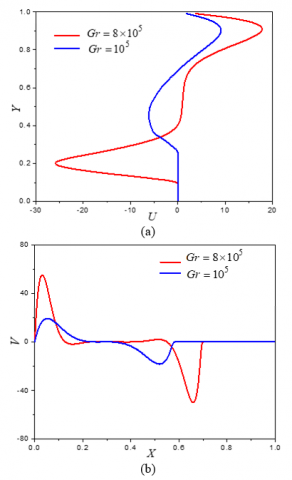Figure 7. Variations of the vertical and horizontal dimensionless velocity U and V at X=0.5 and Y=0.5

## fig.8.pngFigure 8. Average Nusselt number $\overline{Nu}$ along the hot and cold vertical walls for different Grashof number: (a) Gr=105, (b) Gr=8×105

Figure 8 shows the change in the average Nusselt number $\overline{Nu}$ with non-dimensional time. The average Nusselt number is plotted along the hot and cold vertical walls, for the two numbers of Grashof, Gr=105 and Gr=8×105. These figures illustrate the exchange of energy across the two walls and show that the attainment of thermal equilibrium is when the time exceeds τ>10 for Gr=105 and τ>3.9 for Gr=8×105. These results are verified. In fact, when the Grashof number

increases, the elapsed time for arriving at the established steady state decreases following the important energy exchanged. It is also noted that the Nusselt number depends on the Grashof number. $\overline{Nu}$ also follows a high value compared to the previous one. $\overline{Nu}$=7.595 for Gr=105 and $\overline{Nu}$=14.640 for Gr=8×105.

5.2 Effect of aspect ratio

In this numerical study, the influence of the aspect ratio on heat transfer and the structure of the flow for the phenomenon of solidification in rectangular cavity is examined for a fixed Grashof number Gr=3.6×105.

For the steady state regime, the structure of the isotherms coupled with dimensionless streamlines are illustrated for different aspect ratios: A=0.5, A=0.75, A=1 and A=2. The Isotherms coupled to dimensionless streamlines are shown schematically in Figure 9.

For small values of aspect ratio (A=0.5, A=0.75), unicellular structure located at the center of the liquid region of the cavity is observed. For this case, heat transfer is principally conductive.

## fig.9.png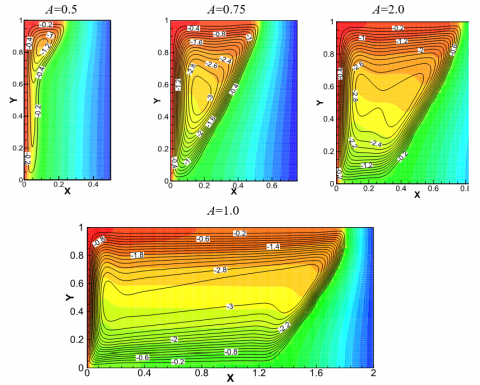Figure 9. Temperature distribution coupled with streamlines for different aspect ratio A

The increase of the aspect ratio from 1 to 2 causes a widening on the unicellular structure of the flow to a structure containing two cells: a main cell located at the center of the liquid part and other more or less small internal arranged. These cells thus generate a recirculation movement and a large convective transport as the aspect ratio (A) becomes large.

These processes can be explained by the increase of the flow, which leads the particles to decrease their course, favoring the transfer of the less convective towards the dominant natural convection. Indeed the impact of the increase in aspect ratio generates the deformation of the isotherms, especially at the interface. In the liquid zones, the curvatures of the isotherms, mainly depend of the distance of the two hot and cold walls or according to the increase of the aspect ratio (A), illustrating the important effect of natural convection. Otherwise, it can also be noted that the aspect ratio (A) has a direct influence on the effect of the structure of the flow. It generates a large convective flow rate in the fluid zone, which directly results in a marked deformation in the bottom of the cavity.

The increase of A has a similar influence as a Grashof number, making the same consequences and favoring the convective transfer in the liquid part in the cavity which may be the dominant type of transfer.

## fig.10.png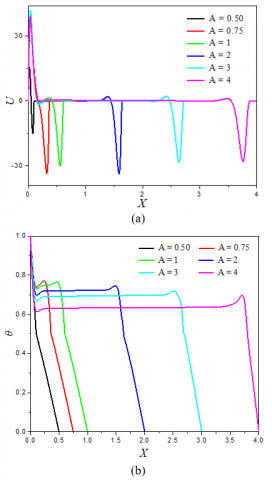Figure 10. Profiles of horizontal velocity (a) and temperature (b) in the middle of the cavity for different aspect ratio A

Figures 10 a-b shows the variations in function of the spatial coordinates of the vertical component U of dimensionless velocity, and dimensionless temperature θ. It appears that these profiles have the same paces. It is also noted that for the different aspect ratio A (0.5, 0.75, 1, 2, 3, 4), the velocity component U changes directions. This can be explained by the important horizontal temperature gradients which generates significant convective motions within the fluid. This explains the increase in the heat transfer rate by natural convection, forced by increasing the aspect ratio from of 0.5 to 4.

6. Conclusions

A numerical study has been developed on heat transfer during a liquid/solid phase change of a fluid (water) inside a rigid-walled rectangular enclosure. The vertical walls are submitted to hot Th and cold Tc temperatures, which surround the melting temperature Tm, (Tc<Tm<Th). In addition, the horizontal walls are adiabatic.

The finite volume method with an enthalpy formulation was used to discretize the equations governing the system, taking into account of conductive and convective transfer modes, and phase change heat transfer of a PCM enclosed in a cavity.  The validation of calculation program was made with experimental and numerical works mentioned in the literature. Different materials have been considered and a good agreement was obtained.

The effects of natural convection and the aspect ratio on solidification of water (PCM) contained in a rectangular cavity were investigated numerically. The results obtained showed that the kinetics of solidification is greatly influenced by theses parameters. The study also shows that physical approach based on a purely conductive model remains limited to cases of low values of the Grashof number.

The present study can be extended to take into account of the effect of the cavity inclination under stationary and non-stationary thermal conditions and phase change materials. Additionally, in the similar manner, the melting phenomenon can be analyzed.

Nomenclature
 A aspect ratio C dimensionless specific heat c f specific heat, J/kg.K liquid fraction Gr Grashof number H height of the cavity, m h mass enthalpy, J/kg $\hbar$ dimensionless enthalpy g gravitational acceleration, m/s2 K dimensionless thermal conductivity k thermal conductivity, W/m.K L length of the cavity, m ℓ latent heat of solidification, J/kg Pr Prandtl number P dimensionless pressure p pressure, Pa S source term T temperature, K t time, s U x dimensionless velocity u x velocity, m/s V y dimensionless velocity υ y velocity, m/s X dimensionless x coordinate Y dimensionless y coordinate Greek symbols a thermal diffusivity, m2. s-1 b thermal expansion coefficient, K-1 μ dynamic viscosity, kg. m-1.s-1 ν kinematic viscosity, m2.s-1 ρ mass density, kg. m-3 τ dimensionless time θ dimensionless temperature Subscripts 0 initial c cold h hot l liquid m solidification r reference s solid
References

 Gupta A, Mathie R, Markides C. (2014). An experimental and computational investigation of a thermal storage system based on a phase change material: Heat transfer and performance characterization. Computational Thermal Sciences 3(4): 341-359. https://doi.org/10.1615/.2014011117

 Talati F, Taghilou M. (2015). Lattice Boltzmann application on the PCM solidification within a rectangular finned container. Applied Thermal Engineering 83(C): 108-120. https://doi.org/10.1016/j.applthermaleng.2015.03.017

 Elnajjar E. (2017). Using PCM embedded in building material for thermal management: Performance assessment study. Energy and Building 151: 28-34. https://doi.org/10.1016/j.enbuild.2017.06.010

 Esapoura M, Hamzehnezhadb A, Darzic AA, Jourabiand M. (2018). Melting and solidification of PCM embedded in porous metal foam in horizontal multi-tube heat storage system. Energy Conversion and Management 171: 398-410. https://doi.org/10.1016/j.enconman.2018.05.086

 Sheikholeslami M. (2018). Numerical modeling of nano enhanced PCM solidification in an enclosure with metallic fin. Journal of Molecular Liquids 259: 424-438. https://doi.org/10.1016/j.molliq.2018.03.006

 Zarajabad OG, Ahmadi R. (2018). Numerical investigation of different PCM volume on cold thermal energy storage system. Journal of Energy Storage 17: 515-524. https://doi.org/10.1016/j.est.2018.04.013

 Gorzin M, Hosseini MJ, Ranjbar AA, Bahrampoury R. (2018). Investigation of PCM charging for the energy saving of domestic hot water system. Applied Thermal Engineering 137: 659-668. https://doi.org/10.1016/j.applthermaleng.2018.04.016

 Zheng H, Wang C, Liu Q, Tian Z, Fan X. (2018). Thermal performance of copper foam/paraffin composite phase change material. Energy Conversion and Management 157: 372-381. https://doi.org/10.1016/j.enconman.2017.12.023

 Teggar M, Mezaache E, Benchatti A, Zeghmati B. (2010). Comparative study of heat transfer during solidification of phase change materials inside three different capsules. International Journal of Heat and Technology 28: 19-23. https://doi.org/10.18280/ijht.280204

 Teggar M, Mezaache E. (2012). Numerical investigation of total solidification time of a liquid phase change material enclosed in rectangular cavities. International Review of Physics 6(2): 158-164.

 Teggar M, Mezaache E. (2013). Numerical investigation of a PCM heat exchanger for latent cool storage. Energy Procedia 1310-1319. https://doi.org/10.1016/j.egypro.2013.07.149

 Kim S, Anghaie S, Chen GA. (2001). fixed-grid two-phase numerical model for convection-dominated melting and solidification. Proc First MIT Conference on Computational Fluid and Solid Mechanics, pp. 12-14.

 Voller VR, Prakash C. (1987). A fixed grid numerical modelling methodology for convection-diffusion mushy region phase-change problems. Int. J. Heat Mass Transf. 30: 1709-1719. https://doi.org/10.1016/0017-9310(87)90317-6

 Michalek T, Kowalewski TA. (2003). Simulations of the water freezing process. Numerical Benchmarks Task Quarterly 7(3): 389-408.

 Wu M, Liu L, Ding J. (2017). An enthalpy method based on fixed-grid for quasi-steady modeling of solidification/melting processes of pure materials. Int. J. Heat Mass Transf. 108: 1383-1392. https://doi.org/10.1016/j.ijheatmasstransfer.2017.01.018

 Murakami H. (1993). Modeling of turbulent flow heat transfer and solidification in a twin roll caster. PhD thesis, McGill University, Canada.

 Patankar SV. (1980). Numerical heat transfer and fluid flow. MacGrawHill.

 Beckermann C, Viskanta R. (1989). Effect of solid subcooling on natural convection melting of a pur metal. International Journal of Heat and Mass Transfer 111: 416-423. https://doi.org/10.1115/1.3250693

 Cerimele MM, Mansutti D, Pistelle F. (2002). Numerical modeling of liquid/solid phase transitions analysis of gallium melting test. Computers and Fluids 31: 437-451. https://doi.org/10.1016/S0045-7930(01)00062-7

 Bouabdallah S. (2006). Etude de l’instabilité hydrodynamique et thermique lors d’un changement de phase avec et sans champ magnetique, Magister thesis, Universite Mentouri Constantine, Algeria.